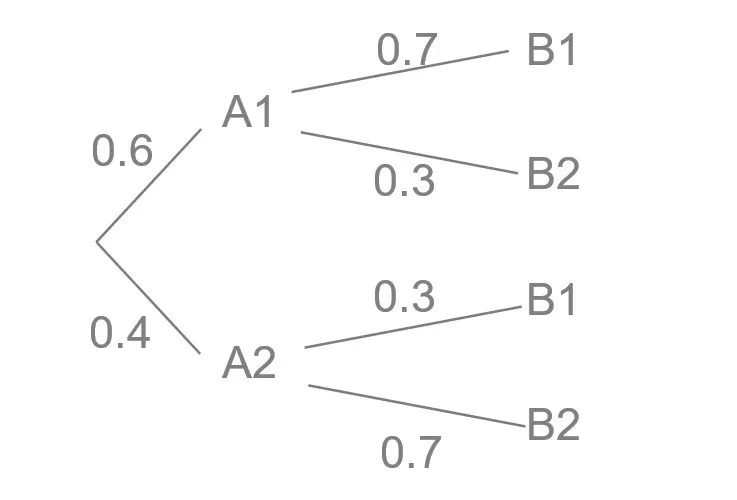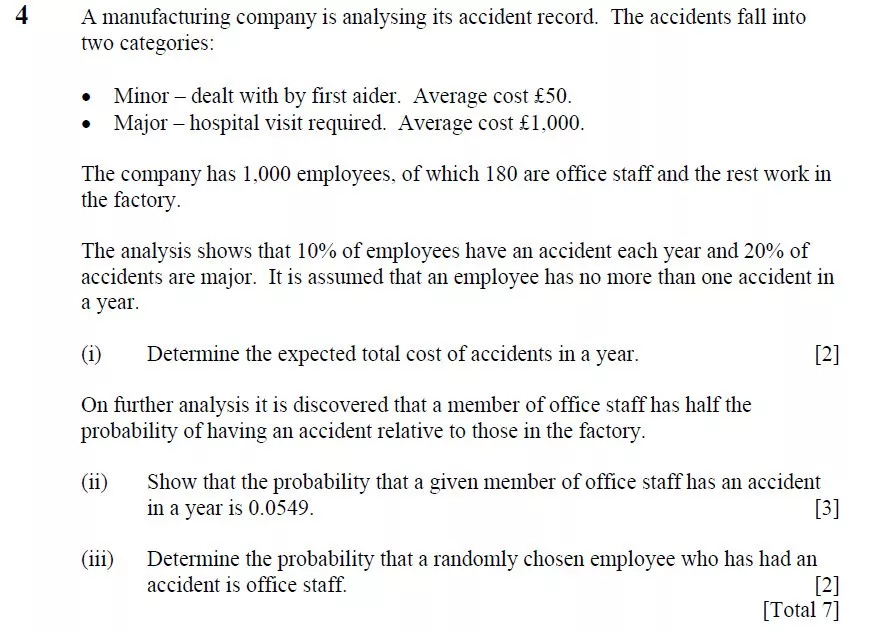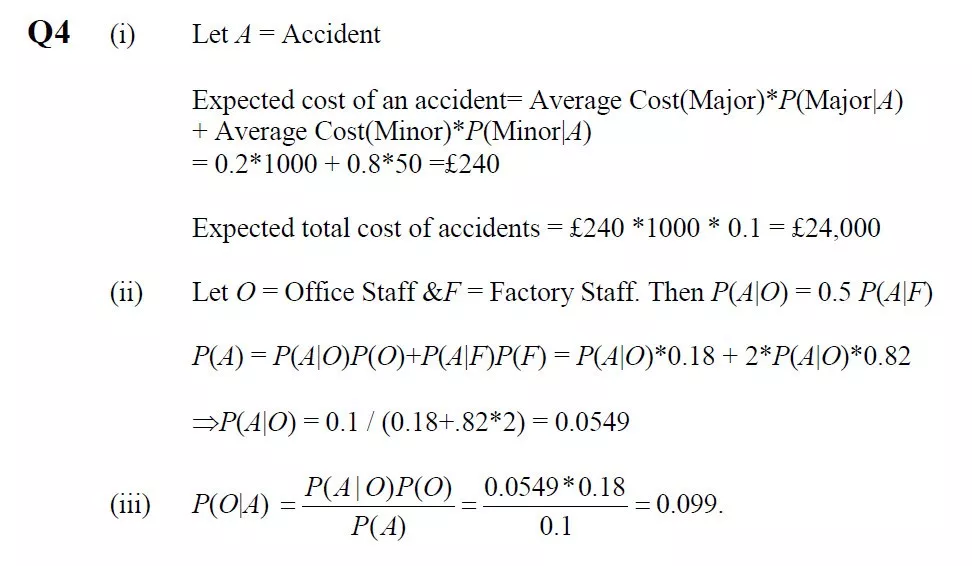### 场景假定

• 其一，Jackie喜欢这个姑娘，因此寤寐思服。
• 其二，Jackie不喜欢这个姑娘，梦见她纯属偶然。

### 设定各事件的概率### 计算条件概率

Jackie在各情形下梦见这个姑娘的概率：

$P\left(B_{1}\right)=0.6 * 0.7+0.4 * 0.3=0.54$

Jackie喜欢并且梦见这个姑娘的概率：

$P\left(A_{1} B_{1}\right)=0.6 * 0.7=0.42$

$P\left(A_{1} | B_{1}\right)=\frac{P\left(A_{1} B_{1}\right)}{P\left(B_{1}\right)}=\frac{7}{9}$

$P\left(A_{2} | B_{1}\right)=\frac{P\left(A_{2} B_{1}\right)}{P\left(B_{1}\right)}=\frac{2}{9}$

### 联想到极大似然估计

“最像”就是“极大似然”的意思。这就暗含了极大似然估计估计的思想：已经发生的事情，就是最可能会发生的。

### 习题We may assume that GuNiang exists first.